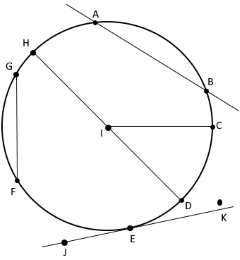# Circles calculation

Description for the calculation of circles

## Circle Definition

A circle is an endless number of points equidistant from a given point. This given point is the center of the circle.

If you draw a segment from the center to any point on the circle, that segment is called radius. Each given circle has an infinite number of radiuses.

The next figure shows some more segments and lines related to circles• The center of the preceding circle is the point $$I$$

• The segment that connect point $$I$$ and point $$C$$ is the radius $$IC$$

• The segment $$HD$$ is the diameter. The diameter connects two points on the circle and passes throuth the center. The length is twice the length of the radius.

• The diameter is a special type of segment or chord that connects two points on a circle. Another example is the chord $$GF$$.

The circle in Figure above has two more lines.

• $$AB$$ is a secant, which is a line that intersects a circle at two points. Secants are both inside and outside of a circle

• $$JK$$ is a tangent, which is a line that intersects a circle at exactly one point. That point of intersection is called the point of tangency

## Circle Calculation

The following table shows the formulas for calculating the properties of a circle

#### Calculate Radius $$r$$ of a circle$$r = \sqrt{A / π}$$

#### Calculate Area $$A$$ of a circle$$A = r^2 · π$$

$$A = d^2 · π / 4$$

#### Calculate Perimeter $$P$$ of a circle$$P = d · π$$

#### Calculate Diameter $$d$$ of a circle$$d = 2 · r$$

$$d = \sqrt{4 · A / π}$$